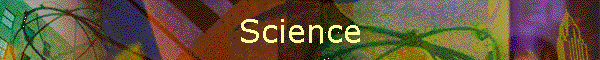Back ] Up ] Next ]

UNIFIED SCIENCE THEORY

First, take Einstein’s linear continuous function which relates energy and mass, and integrate it with respect to time, which then should represent Universe (the area of all mass and energy through time). Over time it is true that universe grows exponentially, so to integrate we should first multiply by an integrating factor, and make this factor an exponential growth notation.

Take this equation and differentiate it with respect to energy, since we all know energy as entropy is ever increasing. Energy tends to radiate, and heat tends to dissipate, there is a constant rate of change from matter to energy caused by internal energies seen in radiation and radioactive decay.

Now differentiate it again with respect to mass, since it is the configurations and formations of mass occurring only in LIFE, and NATURE that allow the natural perpetual rate of change from matter to energy which is seen and known as life.

Finally it is differentiated again with respect to Time, to express the Acceleration of the first two steps. This Acceleration he described as Evolution, and the physical processes of the Accelerated changes from Matter to Energy which is only occurring in the nervous systems of an Animal, and only Maximized in the mental conceptual processes of Men’s Minds. This final differentiation is the greatest unification since it expresses the accelerated changes of matter to energy which is an internal fact for all objects which display gravity, as the Weak Force or the Strong Force. Further this Acceleration is what causes these forces, just as the accelerated processes occurring only in the minds of men display themselves as similar forces of Love and Coincidence. Fate is thus defined by this acceleration in the minds of men, who with knowledge can utilize this force and define it.

Now we can integrate what is left and find that the area has increased, proving the facts of evolution, expressed by the equations.